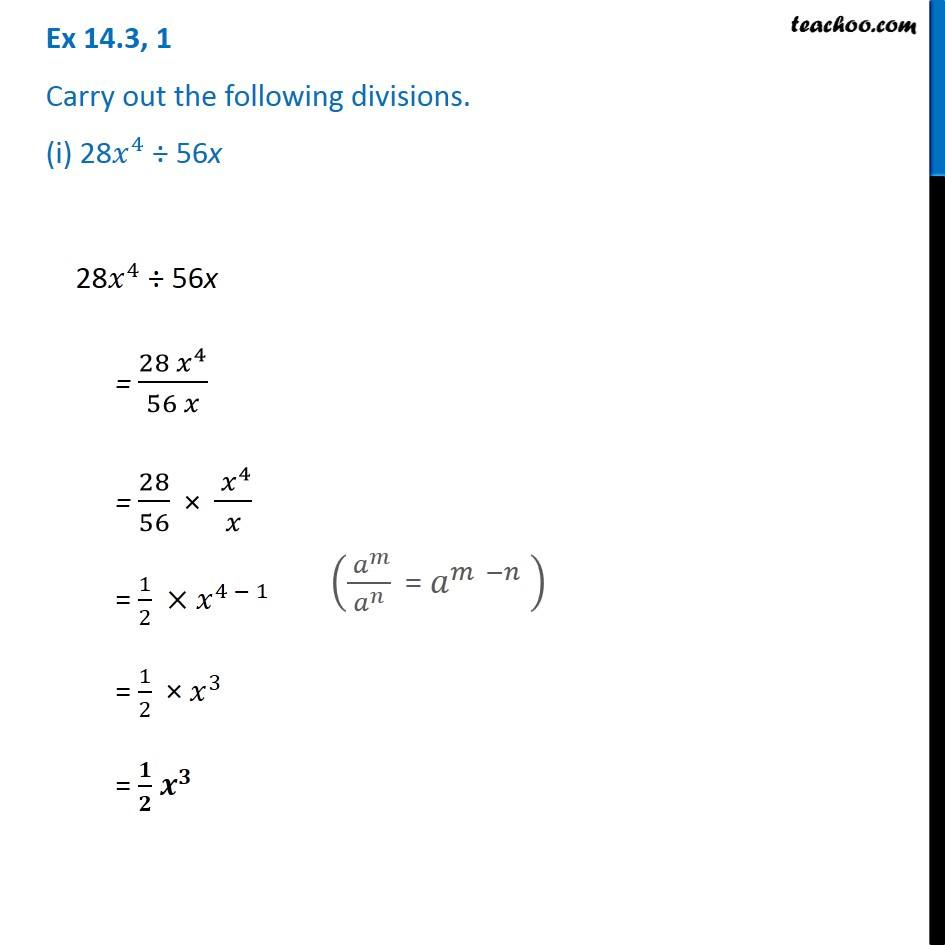Subscribe to our Youtube Channel - https://you.tube/teachoo

1. Chapter 14 Class 8 Factorisation
2. Serial order wise
3. Ex 14.3

Transcript

Ex 14.3, 1 Carry out the following divisions. (i) 28𝑥^4 ÷ 56x 28𝑥^4 ÷ 56x = (28〖 𝑥〗^4)/(56 𝑥) = 28/56 × 〖 𝑥〗^4/𝑥 = 1/2 ×𝑥^(4 − 1) = 1/2 × 𝑥^3 = 𝟏/𝟐 𝒙^𝟑 28𝑥^4 ÷ 56x = (28〖 𝑥〗^4)/(56 𝑥) = 28/56 × 〖 𝑥〗^4/𝑥 = 1/2 ×𝑥^(4 − 1) = 1/2 × 𝑥^3 = 𝟏/𝟐 𝒙^𝟑 (( 𝑎^𝑚)/𝑎^𝑛 " = " 𝑎^(𝑚 −𝑛) " " )

Ex 14.3